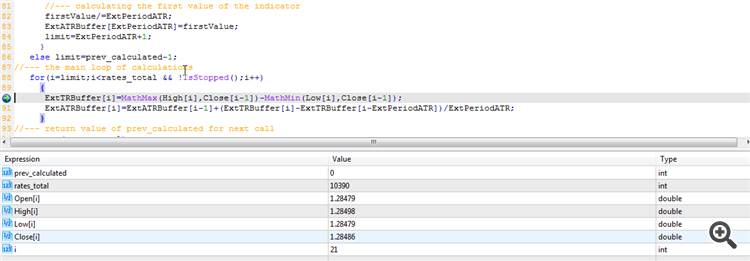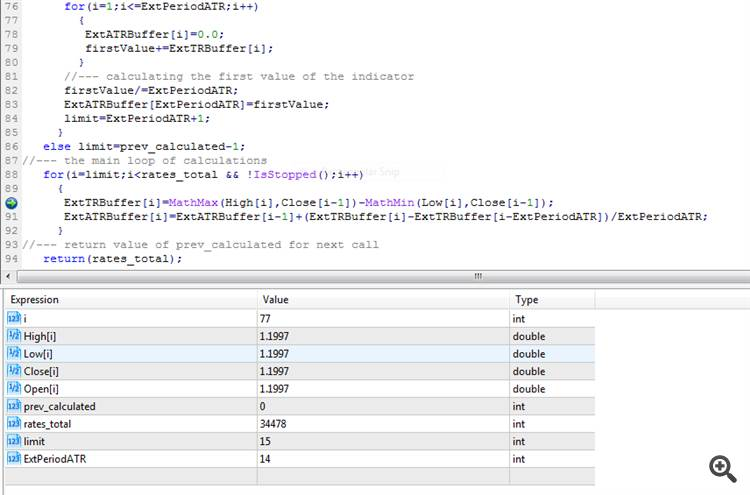oncalculate OHCL same value218

hello guys,

I'm making  an indicator where I need open close high low of each bar.

the problem is that open[i] == close[i] == high[i] == low[i] so if I need to compute Log(close[i]/open[i]); I always get zero!!

why is that happening?

I've used the debugger on mt5 built in indicators (ex ATR) that uses OHLC and the same equality occurs.

for example ATR uses:

MathMax(High[i],Close[i-1])-MathMin(Low[i],Close[i-1])

where in the debugger I get High[i] == Low[i]

can someone explain?39032

michelino:

hello guys,

I'm making  an indicator where I need open close high low of each bar.

the problem is that open[i] == close[i] == high[i] == low[i] so if I need to compute Log(close[i]/open[i]); I always get zero!!

why is that happening?

I've used the debugger on mt5 built in indicators (ex ATR) that uses OHLC and the same equality occurs.

for example ATR uses:

MathMax(High[i],Close[i-1])-MathMin(Low[i],Close[i-1])

where in the debugger I get High[i] == Low[i]

can someone explain?

Your code is probably buggedWhen a new candle is started you have open[i] == close[i] == high[i] == low[i], if i = index of current candle.218

angevoyageur:
Your code is probably bugged When a new candle is started you have open[i] == close[i] == high[i] == low[i], if i = index of current candle.

why the same is happening in ATR code? I don't think it's bugged since it's mql5 built in indicator

am I using wrongly the debugger?39032

michelino:

why the same is happening in ATR code? I don't think it's bugged since it's mql5 built in indicator

am I using wrongly the debugger?

It's difficult to say, can you provide some code to demonstrate your issue ?218

angevoyageur:
It's difficult to say, can you provide some code to demonstrate your issue ?

here the oncalculate of ATR indicator made by metaquote programmers (I suppose)

int OnCalculate(const int rates_total,
const int prev_calculated,
const datetime &Time[],
const double &Open[],
const double &High[],
const double &Low[],
const double &Close[],
const long &TickVolume[],
const long &Volume[],
{
int i,limit;
//--- check for bars count
if(rates_total<=ExtPeriodATR)
return(0); // not enough bars for calculation
//--- preliminary calculations
if(prev_calculated==0)
{
ExtTRBuffer=0.0;
ExtATRBuffer=0.0;
//--- filling out the array of True Range values for each period
for(i=1;i<rates_total && !IsStopped();i++)
ExtTRBuffer[i]=MathMax(High[i],Close[i-1])-MathMin(Low[i],Close[i-1]);
//--- first AtrPeriod values of the indicator are not calculated
double firstValue=0.0;
for(i=1;i<=ExtPeriodATR;i++)
{
ExtATRBuffer[i]=0.0;
firstValue+=ExtTRBuffer[i];
}
//--- calculating the first value of the indicator
firstValue/=ExtPeriodATR;
ExtATRBuffer[ExtPeriodATR]=firstValue;
limit=ExtPeriodATR+1;
}
else limit=prev_calculated-1;
//--- the main loop of calculations
for(i=limit;i<rates_total && !IsStopped();i++)
{
ExtTRBuffer[i]=MathMax(High[i],Close[i-1])-MathMin(Low[i],Close[i-1]);
ExtATRBuffer[i]=ExtATRBuffer[i-1]+(ExtTRBuffer[i]-ExtTRBuffer[i-ExtPeriodATR])/ExtPeriodATR;
}
//--- return value of prev_calculated for next call
return(rates_total);
}

if in debugging I add a watch on Low[i] and High[i] whatever "i" is they have the same value.

btw you wrote that  i=index of current candle in case H==L==C==O so should I use i-1 to get the previous (formed) candle?39032

michelino:

here the oncalculate of ATR indicator made by metaquote programmers (I suppose)

if in debugging I add a watch on Low[i] and High[i] whatever "i" is they have the same value.

btw you wrote that  i=index of current candle in case H==L==C==O so should I use i-1 to get the previous (formed) candle?

Here a screenshot of a debugging session for ATR indicator, debug point on the first line of the loop :This is for i=21 so an old candle. The current candle in this case has an index of rates_total-1 (10389) and the last closed candle an index of rates_total-2 (10388).

Next is the values for the first tick of a new candle :Here, as you see all values are equal (open, high, low, close). i = rates-total-1 so current (open) candle.

Is that help ?218

angevoyageur:

Here a screenshot of a debugging session for ATR indicator, debug point on the first line of the loop :

This is for i=21 so an old candle. The current candle in this case has an index of rates_total-1 (10389) and the last closed candle an index of rates_total-2 (10388).

Next is the values for the first tick of a new candle :

Here, as you see all values are equal (open, high, low, close). i = rates-total-1 so current (open) candle.

Is that help ?

yes it helps a lot, I don't know what have i done wrong during debugging, but the concept is clear now. I'm going to debug again and let you know if it works.

thanks Alain218

I'm sorry there's something I'm still missing.

the following screenshot is dureing ATR debugging:i=77 is an old completely formed candle but O==C==H==L

and it's the same for all i>14 up to 77 then I stopped clicking

the concept you explained is clear but the output of my debugger is different from yours.

do you have any clue?

data corrupted? bars not formed properly?

what can I check?39032

michelino:

I'm sorry there's something I'm still missing.

the following screenshot is dureing ATR debugging:

i=77 is an old completely formed candle but O==C==H==L

and it's the same for all i>14 up to 77 then I stopped clicking

the concept you explained is clear but the output of my debugger is different from yours.

do you have any clue?

data corrupted? bars not formed properly?

what can I check?

You probably have bad or corrupted data. Do you checked the corresponding chart ? You can also try with other symbol/timeframe.218

angevoyageur:

You probably have bad or corrupted data. Do you checked the corresponding chart ? You can also try with other symbol/timeframe.

yes it is bad data on other pairs everything works fine, even my indicator

thanks a lot